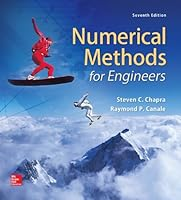# Numerical Methods for Engineers, 7th Edition## Book Description

The seventh edition of Chapra and Canale&#39s Numerical Methods for Engineers retains the instructional techniques that have made the text so successful. Chapra and Canale&#39s unique approach opens each part of the text with sections called &#8220Motivation,“ &#8220Mathematical Background,” and &#8220Orientation” Each part closes with an &#8220Epilogue” containing &#8220Trade-Offs,” &#8220Important Relationships and Formulas,” and &#8220Advanced Methods and Additional References.” Much more than a summary, the Epilogue deepens understanding of what has been learned and provides a peek into more advanced methods. Helpful separate Appendices. &#8220Getting Started with MATLAB” and &#8220Getting Started with Mathcad” which make excellent references.

Numerous new or revised problems are drawn from actual engineering practice. The expanded breadth of engineering disciplines covered is especially evident in these exercises, which now cover such areas as and biomedical engineering. Excellent new examples and case studies span all areas of engineering giving students a broad exposure to various fields in engineering.

Users will find use of files for many popular software packages, specifically MATLAB&#174, Excel® with , and Mathcad&#174. There is also material on developing MATLAB® m-files and macros.

CHAPTER 1 and Engineering Problem Solving
CHAPTER 2 and Software
CHAPTER 3 Approximations and Round-Off Errors
CHAPTER 4 Truncation Errors and the Taylor Series
CHAPTER 5 Bracketing Methods
CHAPTER 6 Open Methods
CHAPTER 7 Roots of Polynomials
CHAPTER 8 Case Studies: Roots of Equations
CHAPTER 9 Gauss Elimination
CHAPTER 10 LU Decomposition and Matrix Inversion
CHAPTER 11 Special Matrices and Gauss-Seidel
CHAPTER 12 Case Studies: Linear Equations
CHAPTER 13 One-Dimensional Unconstrained Optimization
CHAPTER 14 Multidimensional Unconstrained Optimization
CHAPTER 15 Constrained Optimization
CHAPTER 16 Case Studies: Optimization
CHAPTER 17 Least-Squares Regression
CHAPTER 18 Interpolation
CHAPTER 19 Fourier Approximation
CHAPTER 20 Case Studies: Curve Fitting
CHAPTER 21 Newton-Cotes Formulas
CHAPTER 22 Integration of Equations
CHAPTER 23 Numerical Differentiation
CHAPTER 24 Case Studies: Numerical Integration and Differentiation
CHAPTER 25 Runge-Kutta Methods
CHAPTER 26 Stiffness and Multistep Methods
CHAPTER 27 Boundary-Value and Eigenvalue Problems
CHAPTER 28 Case Studies: Ordinary Differential Equations
CHAPTER 29 Finite Difference: Elliptic Equations
CHAPTER 30 Finite Difference: Parabolic Equations
CHAPTER 31 Finite-Element Method
CHAPTER 32 Case Studies: Partial Differential Equations

APPENDIX A: THE FOURIER SERIES
APPENDIX B: GETTING STARTED WITH MATLAB
APPENDIX C: GETTING STARTED WITH MATHCAD